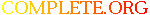Complete.Org: Mailing Lists: Archives: freeciv-dev: August 2004: [Freeciv-Dev] (PR#9865) CM error# [Freeciv-Dev] (PR#9865) CM error

[Top] [All Lists]

[Date Prev][Date Next][Thread Prev][Thread Next][Date Index] [Thread Index]
 To: per@xxxxxxxxxxx Subject: [Freeciv-Dev] (PR#9865) CM error From: "Jason Short" Date: Mon, 30 Aug 2004 19:14:23 -0700 Reply-to: rt@xxxxxxxxxxx

```<URL: http://rt.freeciv.org/Ticket/Display.html?id=9865 >

> [per - Sun Aug 29 20:10:39 2004]:
>
> Program received signal SIGSEGV, Segmentation fault.
> 0x004b0096 in _int_free () from /lib/tls/libc.so.6
> (gdb) bt
> #0  0x004b0096 in _int_free () from /lib/tls/libc.so.6
> #1  0x004af01b in free () from /lib/tls/libc.so.6
> #2  0x0811c0e3 in build_cache3 (pcity=0x9328680) at cm.c:1189
> #3  0x0811c6e5 in optimize_final (pcity=0x9328680, parameter=0xbfecf7f0,
>     best_result=0xbfecf7a0) at cm.c:1349
> #4  0x0811ce58 in cm_query_result (pcity=0x9328680, parameter=0xbfecf7f0,
>     result=0xbfecf7a0) at cm.c:1500
> #5  0x080653a3 in auto_arrange_workers (pcity=0x9328680) at cityturn.c:228
> #6  0x0810a930 in ai_best_government (pplayer=0x822a1c8) at aihand.c:268
> #7  0x08107991 in ai_data_turn_init (pplayer=0x822a1c8) at aidata.c:281
> #8  0x0804e815 in begin_phase (is_new_phase=false) at srv_main.c:489
> #9  0x0805032a in main_loop () at srv_main.c:1430
> #10 0x080505a6 in srv_main () at srv_main.c:1549
> #11 0x0804a1c0 in main (argc=3, argv=0xbfecfa04) at civserver.c:174
>
> Use attached savegame, /set time -1, /start, *boom*. Savegame added in the
> other RT to this ticket.

I can't reproduce this with current code.  With older code I can get it.
Valgrind gives:

==1489== Invalid write of size 1
==1489==    at 0x812C7B8: ai_data_turn_init (aidata.c:101)
==1489==    by 0x812D1D8: ai_data_get (aidata.c:333)
==1489==    by 0x809EB18: player_load (savegame.c:1432)
==1489==    by 0x80A2CCB: game_load (savegame.c:3220)
==1489==    by 0x8055023: load_command (stdinhand.c:2986)
==1489==    by 0x8059282: handle_stdin_input (stdinhand.c:3222)
==1489==    by 0x80A93A9: handle_readline_input_callback (sernet.c:173)
==1489==    by 0x1B93B560: rl_callback_read_char (in
/lib/libreadline.so.4.3)
==1489==    by 0x80AAE70: sniff_packets (sernet.c:559)
==1489==    by 0x8050994: srv_main (srv_main.c:1590)
==1489==    by 0x804A1FE: main (civserver.c:174)
==1489==  Address 0x1BB7B155 is 3 bytes before a block of size 2 alloc'd
==1489==    at 0x1B904EDD: malloc (vg_replace_malloc.c:131)
==1489==    by 0x804B049: fc_real_calloc (mem.c:79)
==1489==    by 0x812C6BE: ai_data_turn_init (aidata.c:73)
==1489==    by 0x812D1D8: ai_data_get (aidata.c:333)
==1489==    by 0x809EB18: player_load (savegame.c:1432)
==1489==    by 0x80A2CCB: game_load (savegame.c:3220)
==1489==    by 0x8055023: load_command (stdinhand.c:2986)
==1489==    by 0x8059282: handle_stdin_input (stdinhand.c:3222)
==1489==    by 0x80A93A9: handle_readline_input_callback (sernet.c:173)
==1489==    by 0x1B93B560: rl_callback_read_char (in
/lib/libreadline.so.4.3)
==1489==    by 0x80AAE70: sniff_packets (sernet.c:559)
==1489==    by 0x8050994: srv_main (srv_main.c:1590)
==1489==    by 0x804A1FE: main (civserver.c:174)

==1489== Invalid write of size 1
==1489==    at 0x812C7B8: ai_data_turn_init (aidata.c:101)
==1489==    by 0x804F548: main_loop (srv_main.c:489)
==1489==    by 0x805135D: srv_main (srv_main.c:1800)
==1489==    by 0x804A1FE: main (civserver.c:174)
==1489==  Address 0x1C1DDDE5 is 3 bytes before a block of size 2 alloc'd
==1489==    at 0x1B904EDD: malloc (vg_replace_malloc.c:131)
==1489==    by 0x804B049: fc_real_calloc (mem.c:79)
==1489==    by 0x812C6BE: ai_data_turn_init (aidata.c:73)
==1489==    by 0x804F548: main_loop (srv_main.c:489)
==1489==    by 0x805135D: srv_main (srv_main.c:1800)
==1489==    by 0x804A1FE: main (civserver.c:174)
> ==1489==
==1489== Invalid read of size 1
==1489==    at 0x81222A4: ai_eval_buildings (advdomestic.c:85)
==1489==    by 0x812AF93: ai_manage_cities (aicity.c:102)
==1489==    by 0x81301B1: ai_do_last_activities (aihand.c:384)
==1489==    by 0x804F9BF: main_loop (srv_main.c:553)
==1489==    by 0x805135D: srv_main (srv_main.c:1800)
==1489==    by 0x804A1FE: main (civserver.c:174)
==1489==  Address 0x1C1DDDF9 is 15 bytes after a block of size 2 alloc'd
==1489==    at 0x1B904EDD: malloc (vg_replace_malloc.c:131)
==1489==    by 0x804B049: fc_real_calloc (mem.c:79)
==1489==    by 0x812C6BE: ai_data_turn_init (aidata.c:73)
==1489==    by 0x804F548: main_loop (srv_main.c:489)
==1489==    by 0x805135D: srv_main (srv_main.c:1800)
==1489==    by 0x804A1FE: main (civserver.c:174)

I conclude that this crash was caused by the bad write, and not by an
error in the CM code.

jason

```

 [Prev in Thread] Current Thread [Next in Thread]